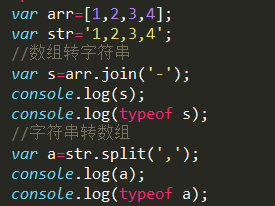JS字符串怎么转数组啊，数组怎么转字符串呢

JS字符串怎么转数组啊，数组怎么转字符串呢JS字符串怎么转数组啊，数组怎么转字符串呢

7个回答

``````// 数组转字符串
var a, b;
a = new Array(0,1,2,3,4);
b = a.join("-");

// 字符串转数组
var s = "abc,abcd,aaa";
ss = s.split(",");// 在每个逗号(,)处进行分解。
``````
``````var str = 'aabbcc';
var strToArr = str.split(''); // [a,a,b,b,c,c]
var  arrToStr = strToArr.join(''); // 'aabbcc'
``````

join(''),实现数组转字符串

```var a, b,c;
a = new Array(a,b,c,d,e);
b = a.join('-'); //a-b-c-d-e 使用-拼接数组元素
c = a.join(''); //abcde

``````split('')实现字符串转数组

``````

var str = 'ab+c+de';
var a = str.split('+'); // [ab, c, de]
var b = str.split(''); //[a, b, +, c, +, d, e]

``````

``````

String[] str = {"abc", "bcd", "def"};
StringBuffer sb = new StringBuffer();
for(int i = 0; i < str.length; i++){
sb. append(str[i]);
}
String s = sb.toString();

char[] data={'a','b','c'};

String s=new String(data);-------仅供参考

。。。。你这思路就不对，什么是字符串转数组？你要想明白，字符串，可以劈开，可以根据下标截，很多方法，只要接收了就是数组，你这话就不对，你的意思是转成数字类型么？2 年多之前 回复js 字符串转单个字符数组

number=12345 将var a=\${number};转成数组aa=1;aa=2;aa=3;aa=4;aa=5;并且迭代数组

JS如何将多维数组转换为带大括号的字符串

javascript中如何将字符数组转为整型数组

Android-转换字符串数组到json

{'type':[{'type_id':'1','type_name':'美容'},{'type_id':'2','type_name':'美发'}]} 这是我传过去的JSON字串。。我想将其转换为数组。 数组格式为row[type_id] 这个值为1 不要之前的那个type，那是表名 请问我该如何处理。。用JAVASCRIPT 或者 JQUERY

Laravel在将数组存储到Json数据库字段时获得“数组到字符串转换”

<div class="post-text" itemprop="text"> <p>I'm trying to save an array with options into a json datafield of my postgres database. I'm working with Laravel 5.5 and I'm using the extension "dimsav/laravel-translatable" for translations.</p> <p>My model Question looks like this: namespace App;</p> <pre><code>use Illuminate\Database\Eloquent\Model; use Dimsav\Translatable\Translatable; class Question extends Model { use Translatable; public \$translatedAttributes = ['options', 'answer']; protected \$casts = [ 'options' =&gt; 'array' ]; } </code></pre> <p>The model QuestionTranslation looks like this:</p> <pre><code>namespace App; use Illuminate\Database\Eloquent\Model; class QuestionTranslation extends Model { public \$timestamps = false; public \$fillable = ['options', 'answer']; </code></pre> <p>}</p> <p>And the store action in the QuestionsController:</p> <pre><code> public function store(Request \$request) { \$question = new Question(); \$options = "test1"; \$options = "test2"; \$question-&gt;answer = 1; \$question-&gt;options = \$options; \$question-&gt;save(); } </code></pre> <p>When I try to store that data I get the error:</p> <pre><code>Illuminate \ Database \ QueryException Array to string conversion (SQL: insert into "question_translations" ("locale", "answer", "options", "question_id") values (en, 1, test1, 18) returning "id") </code></pre> <p>When I use <code>json_encode</code> to cast \$options myself, I can store it without problems. </p> <p>Do you have any idea, why the laravel casting is not working? Maybe because of the translatable extension?</p> </div>

``` var arrData='["2015-06-02 13:23:30","2015-06-02 13:22:30","2015-06-03 13:23:30","2015-06-04 13:23:30","2015-06-05 13:23:30",]'; ``` 我想最终的效果是这个新数组A的值为2015-06-02 13:23:30

<div class="post-text" itemprop="text"> <p>I have try for days, to make my query row as array but haven't success yet. I have 'KD.1','KD.2','KD.3','KD.4','KD.5','KD.1','KD.2','KD.3','KD.4','KD.5' in mysql row and want to make this as array so i will get 10 array rather than 1. Next i will use it to combine with another row that has same pattern. Look like this 0,0,1,1,0,0,1,1,1,0</p> <pre><code>while(\$row = \$result-&gt;fetch_array(MYSQLI_ASSOC)) { \$keys = Array(\$row['listkd']); \$values = Array(\$row['rightval']); \$final_array [\$row['testnis']]= array_combine_(\$keys, \$values); } </code></pre> <p>my function array_combine_ i get from <a href="https://php.net/manual/ru/function.array-combine.php" rel="nofollow noreferrer">here</a></p> <pre><code>function array_combine_(\$keys, \$values){ \$result = array(); foreach (\$keys as \$i =&gt; \$k) { \$result[\$k][] = \$values[\$i]; } array_walk(\$result, function(&amp;\$v){ \$v = (count(\$v) == 1) ? array_pop(\$v): \$v; }); return \$result; } </code></pre> <p>I always get one array rather than ten, so its can't be combine two arrays. What i want the result is like this</p> <pre><code> =&gt; Array ( [KD.1] =&gt; Array (  =&gt; 0  =&gt; 0 ) [KD.2] =&gt; Array (  =&gt; 0  =&gt; 1 ) [KD.3] =&gt; Array (  =&gt; 1  =&gt; 1 ) [KD.4] =&gt; Array (  =&gt; 1  =&gt; 1 ) [KD.5] =&gt; Array (  =&gt; 0  =&gt; 0 ) )  =&gt; Array ( [KD.1] =&gt; Array (  =&gt; 0  =&gt; 0 ) [KD.2] =&gt; Array (  =&gt; 0  =&gt; 1 ) [KD.3] =&gt; Array (  =&gt; 1  =&gt; 1 ) [KD.4] =&gt; Array (  =&gt; 1  =&gt; 1 ) [KD.5] =&gt; Array (  =&gt; 0  =&gt; 0 ) ) </code></pre> </div>

<div class="post-text" itemprop="text"> <p>I need to pass an array passed in Twig as a parameter for a javascript object.</p> <pre><code> \$app-&gt;render("site/home.twig.html", array( "page" =&gt; "home", "trans" =&gt; \$translationfile )); </code></pre> <p>\$translationfile is a value generated from json_decode'ing a JSON file containing translation values.</p> <p>When I attempt to access the part of the file I want to access;</p> <pre><code>var translations = "{{ trans.production }}"; </code></pre> <p>I get "Array" as an answer.</p> <p>I assume there's a way to obtain the array? Do I need to encode it?</p> </div>

js json数据转化成数组问题

ajax返回的数据如何在前台转换为数组

JSON数组如何去除中括号两旁的双引号

"RoomDetailList":"[{\"PSMemo\":\"0\",\"Name\":\"0\",\"Code\":\"4444\",\"ModelNo\":\"4\",\"Num\":\"4\",\"UnitPrice\":\"4\",\"Memo\":\"4\"},{\"PSMemo\":\"0\",\"Name\":\"0\",\"Code\":\"4444\",\"ModelNo\":\"4\",\"Num\":\"4\",\"UnitPrice\":\"4\",\"Memo\":\"4\"}]"

json数据如何转存到数组中来？

Java基础知识面试题（2020最新版）

Linux 会成为主流桌面操作系统吗？

loonggg读完需要3分钟速读仅需 1 分钟大家好，我是你们的校长。我之前讲过，这年头，只要肯动脑，肯行动，程序员凭借自己的技术，赚钱的方式还是有很多种的。仅仅靠在公司出卖自己的劳动时...

MySQL数据库面试题（2020最新版）

Redis核心原理与应用实践

Redis核心原理与应用实践 在很多场景下都会使用Redis，但是到了深层次的时候就了解的不是那么深刻，以至于在面试的时候经常会遇到卡壳的现象，学习知识要做到系统和深入，不要把Redis想象的过于复杂，和Mysql一样，是个读取数据的软件。 有一个理解是Redis是key value缓存服务器，更多的优点在于对value的操作更加丰富。 安装 yum install redis #yum安装 b...

!大部分程序员只会写3年代码

2020阿里全球数学大赛：3万名高手、4道题、2天2夜未交卷

HTTP与HTTPS的区别